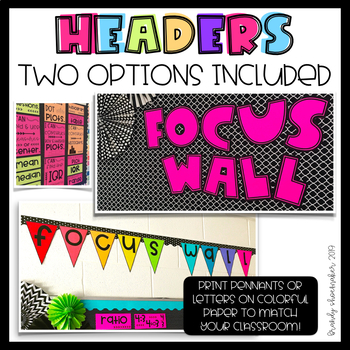# Focus Wall Kit Geometry 6th Grade MathSubject
Resource Type
File Type

PDF

(14 MB|49 pages)
Product Rating
Standards
• Product Description
• StandardsNEW

Math Focus Wall: Geometry

Support your students in building their learning by incorporating a math focus wall into your daily routine! This resource includes the learning target posters, vocabulary cards, and focus wall header options to help you build a math focus wall for your Geometry unit with topics including area of triangles and quadrilaterals, area of complex shapes, representing 3-D shapes with nets, using nets to find surface area, and finding volume of rectangular prisms with fractional edge lengths.

The eye-catching posters and vocabulary cards are designed with clean & crisp black and white that can be printed on the colorful paper/card stock of your choice to match your classroom decor/theme. The modern and playful fonts by Amy Groesbeck and Kimberly Geswein coordinate with many other classroom decor, signs, and resources found on Teachers Pay Teachers. This focus wall kit will fit seamlessly into your sixth grade math classroom!

*SEE PREVIEW* for usage ideas and images showing what is included in this product.

Vocabulary:

• right triangle
• area
• triangle
• polygon
• right
• edge
• volume
• net
• compose
• decompose
• fractional
• rectangular prism
• prism
• pyramid
• formula
• unit cubes
• triangular prism
• pentagonal prism
• triangular pyramid
• V=LWH
• A=BH
• A=LW
• A=1/2BH
• represent
• rectangle
• surface area
• properties
• face
• vertex
• complex shape
• base
• length
• width
• height
• 2-D
• 3-D
• trapezoid
• square pyramid
• cube
• cylinder
• parallelogram
• cone
• rhombus

Learning Targets:

• I can find the area of a triangle.
• I can find the area of a rectangle.
• I can find the area of a quadrilateral.
• I can find the area of a complex shape.
• I can decompose complex shapes to find the area.
• I can solve problems involving area.
• I can solve problems involving surface area.
• I can represent 3-D shapes with a net.
• I can use a net to find the surface area of a 3-D shape.
• I can solve problems involving volume.
• I can use the volume formulas to solve problems.
• I can show that the formula for volume works.
• I can find the volume of a rectangular prism.

Standards Alignment:
CCSS
6.G.1

6.G.2

6.G.4

TEKS

6.8 A, B, C, D

Preview and thumbnail images include fonts from Amy Groesbeck and Kimberley Geswein.

Represent three-dimensional figures using nets made up of rectangles and triangles, and use the nets to find the surface area of these figures. Apply these techniques in the context of solving real-world and mathematical problems.
Find the volume of a right rectangular prism with fractional edge lengths by packing it with unit cubes of the appropriate unit fraction edge lengths, and show that the volume is the same as would be found by multiplying the edge lengths of the prism. Apply the formulas 𝘝 = 𝘭 𝘸 𝘩 and 𝘝 = 𝘣 𝘩 to find volumes of right rectangular prisms with fractional edge lengths in the context of solving real-world and mathematical problems.
Find the area of right triangles, other triangles, special quadrilaterals, and polygons by composing into rectangles or decomposing into triangles and other shapes; apply these techniques in the context of solving real-world and mathematical problems.
Total Pages
49 pages
Does not apply
Teaching Duration
3 Weeks
Report this Resource to TpT
Reported resources will be reviewed by our team. Report this resource to let us know if this resource violates TpT’s content guidelines.